# 3-2: Structures: Math and Minipage

In this lesson we describe a few more commands that are commonly used to typeset mathematics in a structured way, or with symbols on top. At the end, we describe the minipage environment, which lets you typeset content in a fixed width region.

## Math Environments

At this point we have discovered a vast array of mathematical symbols and constructs to typeset mathematical expressions and equations beautifully.  Now we introduce a set of mathematical environments that will affect the layout and organization of the math included in our documents.

### The equation Environment

The equation environment is very similar to $$display mode$$, with one major difference (which the following environment also shares): any expression made with the equation environment will be numbered.

Example: \begin{equation}a^2+b^2=c^2\end{equation} would display: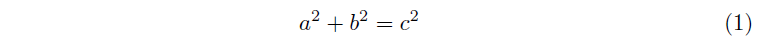This number can be used as a reference anywhere else in the document, which will be described in the next lesson.

If for some reason you did not wish to number the mathematical expression within an equation environment, you can place an asterisk after equation in the \begin{equation*} and \end{equation*} commands.

### The align Environment

With the exception of the array environment, every math environment we have worked with so far has only accommodated one line of mathematics.  The align environment is able to support multiple lines of math, each of which can be divided into two columns.  The columns are justified {rl} and math is entered exactly how one would enter math into an array. The align environment requires the amsmath package.

The code below and the corresponding image it generates show a simplification of the quadratic equation when $$a=1,b=-3,c=2$$.

\begin{align}
x & = \frac{3\pm\sqrt{(-3)^2-4(1)(2)}}{2(1)}\\
& = \frac{3\pm\sqrt{9-8}}{2}\\
& = \frac{3\pm\sqrt{1}}{2}\\
& = \frac{3\pm1}{2}\\
& = 2\textrm{ or } 1
\end{align}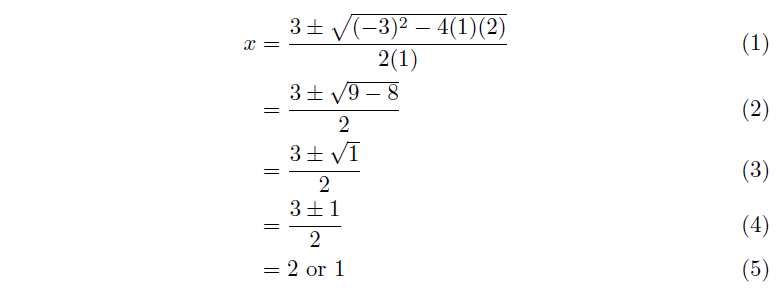You’ll notice that the align environment also provides automatic labelling, but for every line of the environment.  To prevent this labelling from occuring, you can place an asterisk inside of the \begin{align*} and \end{align*} commands as we did before.  Alternatively, you can use the command \notag immediately before the newline \\ on a particular line to prevent that line from being numbered.

We mentioned the environment can be divided into two columns; however, it can also be divided into more than two columns too.  Note what the code below gives us when we typeset it:

\begin{align*}
a & = 1  &  b & = 2 \\
c & = 3  &  d & = 4
\end{align*}

\begin{align*}a&=1\qquad\qquad&\qquad\qquad b&=2\\c&=3&d&=4\end{align*}

So in reality, the real column set up for the align environment is {rlrlrl...}, with pairs of columns being equally spaced across the page.  To have the outer pairs of columns pushed to the edges of the pages, use the flalign environment.

In some cases, you may not want the {rlrlrl...} columns implicit in align. In these cases you may want to investigate the array environment, or the older eqnarray command, which has implicit {rcl} columns and different spacing.

### The cases Environment

The cases environment is an extremely useful math environment that typesets several rows with a brace.  Its purpose is best illustrated by one of the most common situations it’s used for, piecewise equations:

$|x| = \begin{cases} x & : x \ge 0 \\ -x & : otherwise \end{cases}$$|x| = \begin{cases} x & : x \ge 0 \\ -x & : \textrm{otherwise} \end{cases}$

Don’t feel that this environment is limited to being used in just this kind of a situation.  It may very well be you want to group a set of equations, or something of that nature.  Keep an open mind, and always remember all the tools you have at your disposal.

### \overbrace{} and \underbrace{}

Similar to cases, we can rotate the brace and use it to point at or describe particular parts of an expression.  Below, we use an \underbrace{} to illustrate collecting like terms.

\begin{align*}
3x^2 + 6x + 5 - 3x - 12 - x^2 - 7
& = \underbrace{3x^2-x^2} + \underbrace{6x-3x} + \underbrace{5-12-7} \\
\end{align*}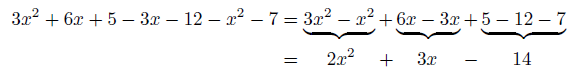For this case, we had to manually space the second line of the align* environment using our math mode spacing commands, along with some trial and error.  There is a way to easily center text over or under an overbrace or an underbrace; use superscripts (^) and subscripts (_).

$5^{73}=\overbrace{5\times5\times\cdots\times5}^{73\textrm{ terms}}$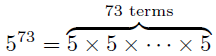## An x Walks Into a Bar

Frequently, you create new variable symbols in mathematics by altering old ones. We give some example here. This is not an exhaustive list, just the ones we think are most likely to be useful to you.

• $$\overline{x}$$ (e.g., expected value):  \overline{x} or use the compact \bar{}
• $$x’$$ (e.g., value after change, or derivative):  x'
• $$x’\!\,’$$:  x''
• $$\dot{v}$$ (e.g., derivative):  \dot{v}
• $$\ddot{v}$$:  \ddot{v}
• $$\vec{v}$$: (e.g., vector):  \vec{v} or use the long \overrightarrow{v}
• $$\widehat{x}$$:  \widehat{x} or use the compact \hat{}
• $$\widetilde{x}$$:  \widetilde{x} or use the compact \tilde{}

# The minipage Environment

The last part of this lesson is not specifically about math typesetting, but instead about a general mechanism for putting information in a fixed-width environment.

You can think of a minipage environment as a mini, inline page.  It takes a specified width, and the height of the environment is determined by how much space is needed to contain the content placed within the environment.  The syntax for using this environment is the following:

\begin{minipage}[«t,c,or b»]{«width»}
% content here
\end{minipage}

The first (optional) parameter is a single letter placed in square brackets [].  This tells LaTeX how to position the minipage with respect to the text it is inline with (or with respect to other minipages on the same line).  You can align the minipage by the top, centre (default) or bottom.

The second (required) parameter indicates the width of the minipage.  Remember that lengths must have units associated with them (such as 10cm or 2in).

For example,

\begin{minipage}{4.8in}
\item The matchsticks currently form 5 squares. Move two matchsticks so that there are 7 squares. Every matchstick must be a part of at least one square, and you may not break matchsticks. Draw the resulting figure.
\end{minipage}\hspace{0.2in}
\begin{minipage}{1in}
\includegraphics[width=1in]{12.pdf}
\end{minipage}

was used to typeset the following piece of a document: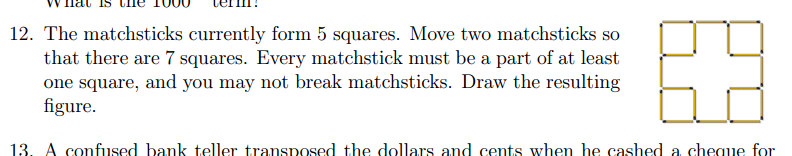In this instance, the author wanted to include images of different sizes next to the text. You can see the whole document here.

### Boxedminipage

You can enhance your minipage by surrounding it with a border. To do this, use the boxedminipage package; and then use the boxedminipage environment in the exact same way that you would use minipage. This package can be useful in an assignment if you want to create a large box for the student to write in. To tweak the border thickness or margins, set the lengths described here.

These are course notes for the University of Waterloo's course Math 600: Mathematical Software.
© 2012—. Written and developed by David Pritchard and Stephen Tosh. Contact (goes to the CEMC)